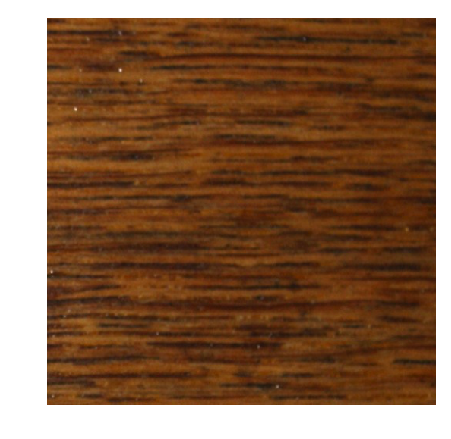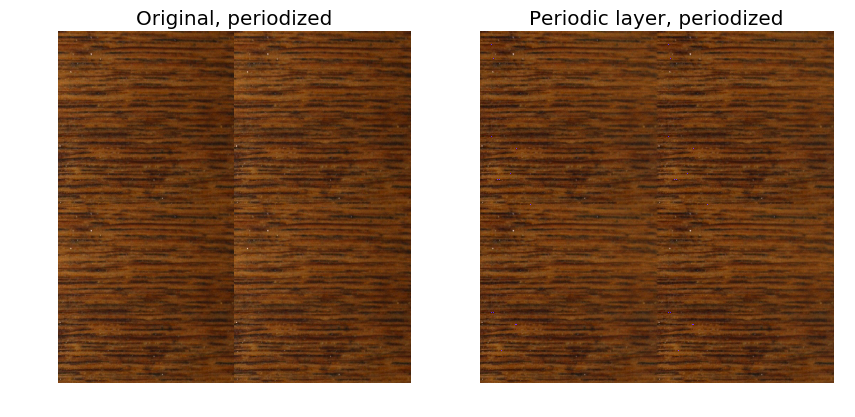# Gaussian Models for Texture Synthesis¶

Important: Please read the installation page for details about how to install the toolboxes. $\newcommand{\dotp}{\langle #1, #2 \rangle}$ $\newcommand{\enscond}{\lbrace #1, #2 \rbrace}$ $\newcommand{\pd}{ \frac{ \partial #1}{\partial #2} }$ $\newcommand{\umin}{\underset{#1}{\min}\;}$ $\newcommand{\umax}{\underset{#1}{\max}\;}$ $\newcommand{\umin}{\underset{#1}{\min}\;}$ $\newcommand{\uargmin}{\underset{#1}{argmin}\;}$ $\newcommand{\norm}{\|#1\|}$ $\newcommand{\abs}{\left|#1\right|}$ $\newcommand{\choice}{ \left\{ \begin{array}{l} #1 \end{array} \right. }$ $\newcommand{\pa}{\left(#1\right)}$ $\newcommand{\diag}{{diag}\left( #1 \right)}$ $\newcommand{\qandq}{\quad\text{and}\quad}$ $\newcommand{\qwhereq}{\quad\text{where}\quad}$ $\newcommand{\qifq}{ \quad \text{if} \quad }$ $\newcommand{\qarrq}{ \quad \Longrightarrow \quad }$ $\newcommand{\ZZ}{\mathbb{Z}}$ $\newcommand{\CC}{\mathbb{C}}$ $\newcommand{\RR}{\mathbb{R}}$ $\newcommand{\EE}{\mathbb{E}}$ $\newcommand{\Zz}{\mathcal{Z}}$ $\newcommand{\Ww}{\mathcal{W}}$ $\newcommand{\Vv}{\mathcal{V}}$ $\newcommand{\Nn}{\mathcal{N}}$ $\newcommand{\NN}{\mathcal{N}}$ $\newcommand{\Hh}{\mathcal{H}}$ $\newcommand{\Bb}{\mathcal{B}}$ $\newcommand{\Ee}{\mathcal{E}}$ $\newcommand{\Cc}{\mathcal{C}}$ $\newcommand{\Gg}{\mathcal{G}}$ $\newcommand{\Ss}{\mathcal{S}}$ $\newcommand{\Pp}{\mathcal{P}}$ $\newcommand{\Ff}{\mathcal{F}}$ $\newcommand{\Xx}{\mathcal{X}}$ $\newcommand{\Mm}{\mathcal{M}}$ $\newcommand{\Ii}{\mathcal{I}}$ $\newcommand{\Dd}{\mathcal{D}}$ $\newcommand{\Ll}{\mathcal{L}}$ $\newcommand{\Tt}{\mathcal{T}}$ $\newcommand{\si}{\sigma}$ $\newcommand{\al}{\alpha}$ $\newcommand{\la}{\lambda}$ $\newcommand{\ga}{\gamma}$ $\newcommand{\Ga}{\Gamma}$ $\newcommand{\La}{\Lambda}$ $\newcommand{\si}{\sigma}$ $\newcommand{\Si}{\Sigma}$ $\newcommand{\be}{\beta}$ $\newcommand{\de}{\delta}$ $\newcommand{\De}{\Delta}$ $\newcommand{\phi}{\varphi}$ $\newcommand{\th}{\theta}$ $\newcommand{\om}{\omega}$ $\newcommand{\Om}{\Omega}$

This numerical tour explores texture synthesis using Gaussian random fields. Image synthesis is obtained by drawing an image at random from a random distribution that is learned from an input texture exemplar.

We use here the spot noise model developped in

B. Galerne, Y. Gousseau and J.-M. Morel, Random Phase Textures: Theory and Synthesis, IEEE Transactions on Image Processing, 20(1), pp. 257-267, 2011.

We derive this model as being a Maximamu Likelihood Estimate (MLE) of a stationary Gaussian vector parameters from a single input texture. Although this is not the original derivation of the model, it is stricly equivalent to the method of Galerne et al.

In :
using PyPlot
using NtToolBox

WARNING: Method definition ndgrid(AbstractArray{T<:Any, 1}) in module NtToolBox at /Users/quentin/.julia/v0.5/NtToolBox/src/ndgrid.jl:3 overwritten at /Users/quentin/.julia/v0.5/NtToolBox/src/ndgrid.jl:3.
WARNING: Method definition ndgrid(AbstractArray{#T<:Any, 1}, AbstractArray{#T<:Any, 1}) in module NtToolBox at /Users/quentin/.julia/v0.5/NtToolBox/src/ndgrid.jl:6 overwritten at /Users/quentin/.julia/v0.5/NtToolBox/src/ndgrid.jl:6.
WARNING: Method definition ndgrid_fill(Any, Any, Any, Any) in module NtToolBox at /Users/quentin/.julia/v0.5/NtToolBox/src/ndgrid.jl:13 overwritten at /Users/quentin/.julia/v0.5/NtToolBox/src/ndgrid.jl:13.
WARNING: Method definition ndgrid(AbstractArray{#T<:Any, 1}...) in module NtToolBox at /Users/quentin/.julia/v0.5/NtToolBox/src/ndgrid.jl:19 overwritten at /Users/quentin/.julia/v0.5/NtToolBox/src/ndgrid.jl:19.
WARNING: Method definition meshgrid(AbstractArray{T<:Any, 1}) in module NtToolBox at /Users/quentin/.julia/v0.5/NtToolBox/src/ndgrid.jl:33 overwritten at /Users/quentin/.julia/v0.5/NtToolBox/src/ndgrid.jl:33.
WARNING: Method definition meshgrid(AbstractArray{#T<:Any, 1}, AbstractArray{#T<:Any, 1}) in module NtToolBox at /Users/quentin/.julia/v0.5/NtToolBox/src/ndgrid.jl:36 overwritten at /Users/quentin/.julia/v0.5/NtToolBox/src/ndgrid.jl:36.
WARNING: Method definition meshgrid(AbstractArray{#T<:Any, 1}, AbstractArray{#T<:Any, 1}, AbstractArray{#T<:Any, 1}) in module NtToolBox at /Users/quentin/.julia/v0.5/NtToolBox/src/ndgrid.jl:44 overwritten at /Users/quentin/.julia/v0.5/NtToolBox/src/ndgrid.jl:44.


## Gaussian Modeling of Textures¶

We consider the modeling of textures $f \in \RR^N$ of $N$ pixels using a Gaussian random vector $X \sim \Nn(\mu,\Si)$. Here $\mu \in \RR^N$ is the mean of the distribution and $\Si \in \RR^{N \times N}$ is a symmetric semi-definite positive covariance matrix.

We recall that formally a random vector is a mapping $X : \Om \rightarrow \RR^N$ where $\Om$ is a probalized space.

Texture analysis corresponds to learning both $\mu$ and $\Si$ from a single exemplar texture $f_0 \in \RR^N$. For this learning to be feasible, since the number of free parameters is enormous, additional assumptions on the distribution are required. We suppose here that the texture model is stationary, i.e. all translates $X(\cdot+\tau)$ have the same distribition for all $\tau \in \ZZ^2$ (we assume here periodic boundary conditions for simplicity).

Texture synthesis corresponds to computing a realization $f = X(\xi) \in \RR^N$ where $\xi \in \Om$, from the random vector $X$. Since $X$ is Gaussian distributed, this can be achieved by computing $f = U w+\mu$ where $U \in \RR^{N \times N}$ is any matrix that factorize the covariance as $\Si = UU^*$ and $w$ is a realisation of a random vector of distribution $\Nn(0,\text{Id}_N)$, which is a Gaussian white noise. In the following, this computation is caried over very easily because the factorization of the covariance is explicitely given during the estimation step.

Load a color textured image $f$.

In :
n = 512


Display it.

In :
figure(figsize = (5,5))
imageplot(f);## Periodic + Smooth Image Decomposition¶

To avoid boundary artifact, we replace the original image $f$ by its periodic component $p$, which is computed as detailed in:

L. Moisan, Periodic plus Smooth Image Decomposition, Journal of Mathematical Imaging and Vision, vol 39:2, pp. 161-179, 2011.

The periodic component $p$ is the solution of the folowing linear system

$$\choice{ \Delta p = \Delta_i f \\ \sum_k p(k) = \sum_k f(k) }$$

where $\Delta$ is a finite difference Laplacian with periodic boundary conditions, and $\Delta_i$ is the same Laplacian but with reflecting boundary conditions.

We first extend the original input image by symmetry to obtain $f_e \in \RR^{(n+2) \times (n+2)}$. Note that the extension of a color image is obtained by extending each channel.

In :
z = zeros(1,1,size(f,3))
fe = [z reshape(f[1,:,:], (1,size(f,2),size(f,3))) z;
reshape(f[:,1,:], (size(f,1),1,size(f,3))) f reshape(f[:,end,:], (size(f,1),1,size(f,3)));
z reshape(f[end,:,:], (1,size(f,2),size(f,3))) z];


Compute the inner-Laplacian $d = \Delta_i f$ as the usual Laplacian of the extended image $\Delta f_e$.

In :
laplacian = x -> 4*x - ( circshift(x,(0, 1)) + circshift(x,(1,0)) + circshift(x,(-1,0)) + circshift(x,(0,-1)) )
d = laplacian(fe)
d = d[2:end-1, 2:end-1, :];


We solve the linear system $\Delta p = d$ (assuming now periodic boundary conditions for the Laplacian) using the Fourier transform $$\forall \om \neq 0, \quad \hat p(\om) = \frac{\hat d(\om)}{ \hat U(\om) } \qwhereq \hat U(\om) = 4 - 2\cos\pa{\frac{2 \om_1 \pi}{n}} - 2\cos\pa{\frac{2 \om_2 \pi}{n}},$$ together with the conservation of the mean constraint $$\hat p(0) = \sum_k f(k).$$

Here, the discrete Fourier transform of an image $f \in \RR^{n \times n}$ is defined as $$\forall (\om_1,\om_2) \in \{0,\ldots,n\}^2, \quad \hat p(\om) = \sum_{k_1=0}^{n-1} \sum_{k_2=0}^{n-1} p(k) e^{\frac{2 i \pi}{n} (k_1 \om_1 + k_2 \om_2) }$$ Note that for a color image, this coefficient is a vector in $\RR^3$ obtained by transforming each channel. The Fourier transform is computed in $O(N\log(N))$ operations with the FFT algorithm (for 2-D image, use the fft2 command).

Compute the Laplacian transform map $\hat U(\om)$.

In :
Y = repeat(0:n-1,outer=(1,n))
X=Y'
U = 4 - 2*cos(2*X*pi/n) - 2*cos(2*Y*pi/n);


Inverse the Laplacian.

In :
P = fft(d,(1,2))./repeat(U, outer=(1, 1, size(f,3)))
P[1,1,:] = sum(sum(f,1),2);
p = real(ifft(P,(1,2)));


Compare the periodic tilings of $f_0$ and $f$.

In :
mydisp = x -> [x x; x x]

figure(figsize = (10,10))
imageplot(mydisp(f), "Original, periodized", [1,2,1])
imageplot(mydisp(p), "Periodic layer, periodized", [1,2,2]);Exercise 1

Compare the log of the modulus of the Fourier transforms of the input image $f$ and its periodic component $p$. What do you observe ?

In :
include("NtSolutions/graphics_1_synthesis_gaussian/exo1.jl")